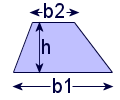# What is the area of a shape?

### Quick Definition:

The area is the two-dimensional amount of space enclosed by a shape. For a square, it's literally the amount of "area" inside the edges of the square. For a three-dimensional object, we use the term surface area to represent the area of the outside surface of the shape.

### Example:

The graphic below is a VERY simple example of the area of a rectangle. Multiplying the side lengths (3 times 4) gives us the area, 12. To think of it visually, 12 squares that are each 1x1 would fit in this area.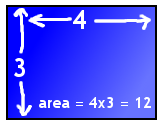### Area Formulas:

Square: $$Area = (Side Length)^2$$

The area of a square is the length of a side, squared.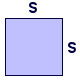Rectangle: $$Area = (Length)*(Width)$$

The area of a rectangle is the length multiplied by the width.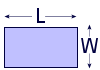Circle: $$Area = \pi*r^2$$

The area of a circle is pi (about 3.14) times the radius squared.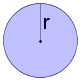Triangle: $$Area = \frac{1}{2}*(Base)*(Height)$$

The area of a triangle is one half the base times the height.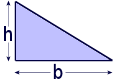Trapezoid: $$Area = \frac{1}{2}*(B_1+B_2)*(Height)$$

The area of a trapezoid is one half the sum of the bases times the height.